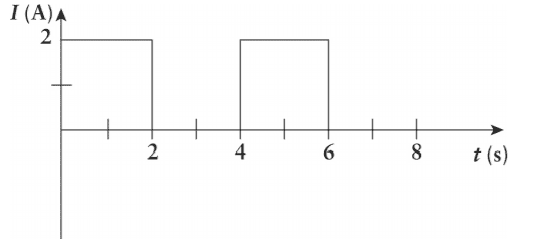# Energy dissipated by resistor problem

• CynicalBiochemist
In summary, the conversation discusses the calculation of energy dissipated by a 10 Ω resistor after 5 seconds. The approach used by the speaker involves finding the average current and using it to calculate the energy. However, the book's approach involves finding the total energy dissipated over the entire 5 seconds and then dividing by the total time. This is because the power generated by the resistor is proportional to the current squared, so the average current cannot be used alone. Additionally, if asked for the power generated by the resistor during the 5 second interval, the correct approach would be to divide the total energy by the total time, as no part of the interval can be neglected.

## Homework Statement

A 10 Ω resistor carries a current that varies as a function of time as shown. How much energy has been dissipated by the resistor after 5 s?a. 40 J
b. 50 J
c. 80 J
d. 120 J

## Homework Equations

I=dq/dt → dq=Idt → Q=∫Idt
P=∇E/∇t and V=IR (Ohm's Law) so P=IV ⇒ P=I^2R

## The Attempt at a Solution

My approach[/B]
For the first 5 seconds, the area under the curve is 6C, which is the total charge that was transferred through the resistor. Thus, the average current I=6C/5s=6/5 A
Since P=I^2R and P=E/∇t ⇒ E=I^2R∇t=(6/5A)^2(10Ω)(5s)= 72J
Clearly this is none of the answer choices, and I don't know where I went wrong.

Book's Approach
The book claims that D is correct, and states:
Since the current was 2A over 3s, the total energy dissipated is
E=(2A)^2(10Ω)(3s)=120J

I genuinely don't understand the book's approach. Power is by definition, the rate of energy transfer (in this case transformation to a different form) with respect to time. Here, the power MUST be computed over the 5 seconds in question, regardless of whether there was 0 current passing through for 2 seconds.
Ultimately, why did the book use 3 seconds as ∇t? Is this allowed, and why is my approach flawed?

#### Attachments

CynicalBiochemist said:
For the first 5 seconds, the area under the curve is 6C, which is the total charge that was transferred through the resistor. Thus, the average current I=6C/5s=6/5 A
Since P=I^2R and P=E/∇t ⇒ E=I^2R∇t=(6/5A)^2(10Ω)(5s)= 72J
Clearly this is none of the answer choices, and I don't know where I went wrong.
You found the average current. But since power is proportional to current squared, to use your approach you'd need the average value of current squared. (Which is not equal to the square of the average current.)

CynicalBiochemist said:
Here, the power MUST be computed over the 5 seconds in question, regardless of whether there was 0 current passing through for 2 seconds.
They did compute the energy over all 5 seconds. Since there was no energy used during the time the current was zero, you can skip that segment.

Doc Al said:
You found the average current. But since power is proportional to current squared, to use your approach you'd need the average value of current squared. (Which is not equal to the square of the average current.)

They did compute the energy over all 5 seconds. Since there was no energy used during the time the current was zero, you can skip that segment.
Thanks Doc. I am starting to understand why their approach works for computing energy. But let's say they asked for the power generated by the resistor for the 5 second interval.
P=120J/3s= 40W ?
I.e., can we again neglect that 2 second segment where no energy was generated? And if so, why?
Btw I'm sorry if I'm asking trivial questions, I've never been good at physics lol.

CynicalBiochemist said:
But let's say they asked for the power generated by the resistor for the 5 second interval.
Don't worry about asking trivial questions! Those are the ones that drive you nuts.•